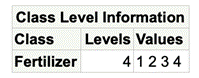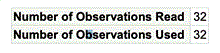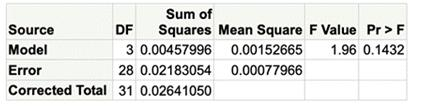One-Way ANOVA

Example Codes:

Objective

Analysis of variance (ANOVA) is a statistical technique used to compare the means of two or more groups of observations or treatments. The continuous variable is the dependent variable and the independent variable is the groups.

The null hypothesis is that there is no difference between the groups. The alternative hypothesis is that there are differences between the groups.

Example code in SAS

data Clover; input Strain \$ Nitrogen @@;

datalines;

3DOK1 19.4

3DOK1 32.6

3DOK1 27.0

3DOK1 32.1

3DOK1 33.0

3DOK5 17.7

3DOK5 24.8

3DOK5 27.9

3DOK5 25.2

3DOK5 24.3

3DOK4 17.0

3DOK4 19.4

3DOK4 9.1

3DOK4 11.9

3DOK4 15.8

3DOK7 20.7

3DOK7 21.0

3DOK7 20.5

3DOK7 18.8

3DOK7 18.6

3DOK13 14.3

3DOK13 14.4

3DOK13 11.8

3DOK13 11.6

3DOK13 14.2

COMPOS 17.3

COMPOS 19.4

COMPOS 19.1

COMPOS 16.9

COMPOS 20.8;

run;

proc anova data = Clover;

class strain;

model Nitrogen = Strain;

run;

Example code in R

clover = data.frame(

strain = c(rep("3DOK1", 5), rep("3DOK5", 5), rep("3DOK4", 5),

rep("3DOK7", 5), rep("3DOK13", 5), rep("COMPOS", 5)),

nitrogen = c(19.4, 32.6, 27.0, 32.1, 33.0, 17.7, 24.8, 27.9, 25.2, 24.3, 17.0,

19.4, 9.1, 11.9, 15.8, 20.7, 21.0, 20.5, 18.8, 18.6, 14.3, 14.4,

11.8, 11.6, 14.2, 17.3, 19.4, 19.1, 16.9, 20.8)

)

summary(aov(nitrogen ~ strain, data = clover))

Outputs

The first table specifies the number of levels and the values of the class variable.The second table shows both the number of observations read and the number of observations used. These values are the same because there are no missing values in for any variable in the model. If any row has missing data for a predictor or response variable, that row is dropped from the analysis.The third part is ANOVA analysis, which is the table here. The column Pr>F indicates the p-value of ANOVA analysis. If it is greater than the significant level, we reject the null hypothesis. So, there are not differences between the groups.  If it is less than the significant level, we fail to reject the null hypothesis. So, there are differences between the groups.